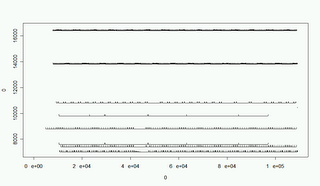## May 11, 2006

### Plot Midi Drum LineFollowing the story of midicsv that converts midi to csv, I wrote some scripts to plot the drum line from an exising midi file.

First, run the midicsv tool to creat the csv file:

Second, run the awk script to filter out everything other than drum beats. The script (filter.awk)

BEGIN{
FS=",";
printf("time,tone,velocity\n");
}
{
if (\$3 ~ /ote/ &&amp;amp; NF==6 && \$4==9)
printf("%s,%s,%s\n",\$2,\$5,\$6);
}
Now, plot the drum line in R (get it from www.r-project.org) Here is the script (midiplot.r)
grid=200;
xmax=max(x\$time);
ymax=max(x\$tone)*grid+200;
plot(0,0,xlim=c(0,xmax),ylim=c(35*grid,ymax));
tone=rep(0,128);
f=factor(x\$tone);
for (i in levels(f)){
t=x\$time[x\$tone==i];
v=x\$velocity[x\$tone==i];
lines(t,as.numeric(i)*grid+v,type="s");
}
Above is the plot result.